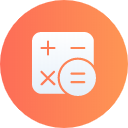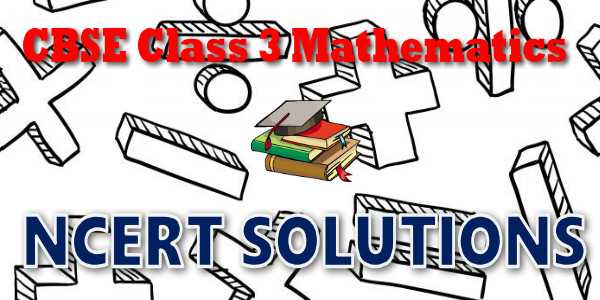No products in the cart.

# CBSE - Class 03 - Mathematics - NCERT Solutions## CBSE, JEE, NEET, CUET

Question Bank, Mock Tests, Exam Papers

NCERT Solutions, Sample Papers, Notes, Videos

## NCERT Solutions for Class 03 Mathematics### Student Subscription

##### Unlock the exclusive content designed for the toppers## myCBSEguide App

Complete Guide for CBSE Students

NCERT Solutions, NCERT Exemplars, Revison Notes, Free Videos, CBSE Papers, MCQ Tests & more.

# NCERT solutions for class 3 Mathematics## Course Structure for class 3 Mathematics

Geometry (16 hrs.)

SHAPES & SPATIAL UNDERSTANDING

• Creates shapes through paper folding, paper cutting.
• Identifies 2-D shapes
• Describes the various 2-D shapes by counting their sides, corners and diagonals.
• Makes shapes on the dot-grid using straight lines and curves.
• Creates shapes using tangram pieces.
• Matches the properties of two 2-D shapes by observing their sides and corners (vertices).
• Tiles a given region using a tile of a given shape.
• Distinguishes between shapes that tile and that do not tile.
• Intuitive idea of a map. Reads simple maps (not necessarily scaled)
• Draws some 3D-objects.

Numbers (42 hrs.)

NUMBER SEQUENCE UPTO 1000

• Reads and writes 3-digit numbers.
• Expands a number w.r.t. place values.
• Counts in different ways – starting from any number.
• Compares numbers.
• Forms greatest and smallest numbers using given digits.

• Adds and subtracts numbers by writing them vertically in the following two cases:

– without regrouping.

– with regrouping.

• Uses the place value in standard algorithm of addition and subtraction.
• Solves addition and subtraction problems in different situations presented through pictures and stories.
• Frames problems for addition and subtraction facts.
• Estimates the sum of, and difference between, two given numbers.

MULTIPLICATION

• Explains the meaning of multiplication (as repeated addition).
• Identifies the sign of multiplication.
• Constructs the multiplication tables of 2, 3, 4, 5 and 10
• Uses multiplication facts in situations.
• Multiplies two digit numbers using standard algorithm and Lattice multiplication algorithm.

DIVISION

• Explains the meaning of division from context of equal grouping and sharing.
• Relates division with multiplication.
• Completes division facts:

– by grouping

– by using multiplication tables.

MENTAL ARITHMETIC

• Adds and subtracts single digit numbers and two digit numbers mentally.
• Doubles two digit numbers mentally (result not exceeding two digits).

Money (5 hrs.)

• Converts Rupee to Paise using play money.
• Adds and subtracts amounts using column addition, and subtraction without regrouping.
• Makes rate charts and bills.

Measurement (21 hrs.)

LENGTH

• Appreciates the need for a standard unit.
• Measures length using appropriate standard units of length by choosing between centimeters and meters.
• Estimates the length of given objects in standard units and verifies by measuring.
• Uses a ruler
• Relates centimeter and meter.

WEIGHT

• Weighs objects using non standard Units.
• Appreciates the conservation of weight.

VOLUME

• Measures and compares the capacity of different containers in terms of non-standard units.
• Appreciates the conservation of volume.

TIME

• Reads a calendar to find a particular day and date.
• Reads the time correct to the hour.
• Sequences the events chronologically.

Data Handling (6 hrs.)

• Records data using tally marks.
• Collects data and represents in terms of pictograph choosing appropriate scale and unit for display through pictographs.
• Draws conclusions from the data by discussing with the teacher.

Patterns (6 hrs.)

• Identifies simple symmetrical shapes and patterns.
• Makes patterns and designs from straight lines and other geometrical shapes.
• Identifies patterns in the numerals for odd and even numbers and in adding odd and even numbers.
• Partitions a number in different ways.
• Identifies patterns in his surroundings
• Identifies patterns in multiplication with, and dividing by 10s.

Here is the list of topics covered under each chapter of class 3 Mathematics NCERT text book.

• 01 Where to Look from
• 02 Fun with Numbers
• 03 Give and take
• 04 Long and short
• 05 Shapes and Designs
• 06 Fun with Give and Take
• 07 Times goes on
• 08 Who is Heavier
• 09 How many Times
• 10 Play with Patterns
• 11 Jugs and Mugs
• 12 Can we Share
• 13 Smart Charts
• 14 Rupees and Paise

## NCERT Solutions for Class 3rd Mathematics

NCERT Solutions Class 3 Maths PDF (Download) Free from myCBSEguide app and myCBSEguide website. Ncert solution class 3 Maths includes text book solutions. NCERT Solutions for CBSE Class 3 Maths have total 14 chapters. Class 3 Maths ncert Solutions in pdf for free Download are given in this website. Ncert Mathematics class 3 solutions PDF and Mathematics ncert class 3 PDF solutions with latest modifications and as per the latest CBSE syllabus are only available in myCBSEguide.

## NCERT Solutions for Class 3## myCBSEguide

Trusted by 1 Crore+ Students## Test Generator

Create papers online. It's FREE.## CUET Mock Tests

75,000+ questions to practice only on myCBSEguide app